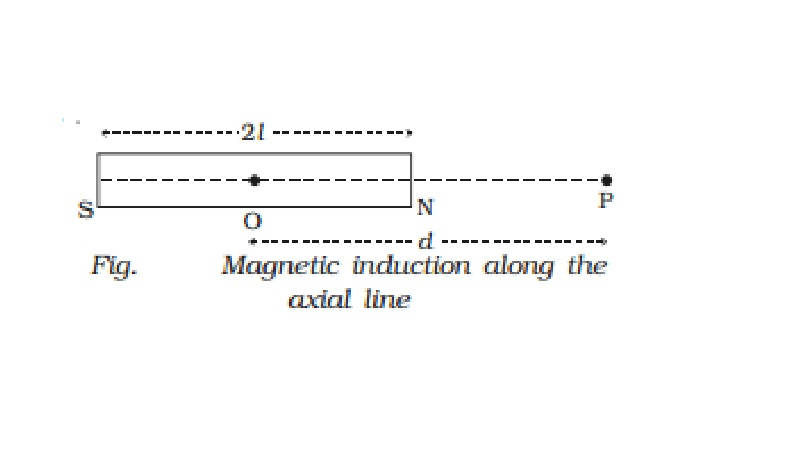Home | | Physics | | Physics | Magnetic induction at a point along the axial line due to a magnetic dipole (Bar magnet)

# Magnetic induction at a point along the axial line due to a magnetic dipole (Bar magnet)NS is the bar magnet of length 2l and of pole strength m. P is a point on the axial line at a distance d from its mid point O (Fig.). According to inverse square law,

Magnetic induction at a point along the axial line due to a magnetic dipole (Bar magnet)

NS is the bar magnet of length 2l and of pole strength m. P is a point on the axial line at a distance d from its mid point O (Fig.).According to inverse square law,

F = ?0/ 4 π . m1m2/d2

Magnetic induction (B1) at P due to north pole of the magnet

B1 = ?0/4 π . m/NP2  along NP

= ?0/4 π . m/(d-l)2  along NP

Magnetic induction (B2) at P due to south pole of the magnet,

B2 = ?0/4 π . m/(SP)2  along PS

B2= ?0/4 π . m/(d+l)2  along PS

Magnetic induction at P due to the bar magnet,

B = B1 ? B2

B = ?0/4 π . 2Md/(d2-l2)2

where M = 2ml (magnetic dipole moment).

For a short bar magnet, l is very small compared to d, hence l 2  is neglected.

B = ?0/4 π . 2Md3

The direction of B is along the axial line away from the north pole.

Coulomb?s inverse square law

Coulomb?s inverse square law states that the force of attraction or repulsion between the two magnetic poles is directly proportional to the product of their pole strengths and inversely proportional to the square of the distance between them.

Study Material, Lecturing Notes, Assignment, Reference, Wiki description explanation, brief detail

Related Topics